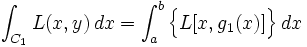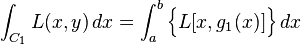# Green's theorem: problem with proof

hi everybody, this is my first post, hope you can help me

check this proof for Green's theorem for a particular case:
http://en.wikipedia.org/wiki/Green's_theorem

rigth after equation (3) you have to calculate the integral\int_{C_1} L(x,y)\, dx = \int_a^b \Big\{L[x,g_1(x)]\Big\}\, dx
but this is an integral of a function calculated on a curve, so there should be also
norm( C1' ) = sqrt( 1^2 + g'(x)^2 )

wikipedia's proof is rigth, I checked it on a book, so where am I wrong?big thanks to all those who will answer!

Last edited:

Suppose you have a real valued function on a plane, $$r:\mathbb{R}^2\to\mathbb{R}$$ and a path on the plane, $$\phi:[a,b]\to\mathbb{R}^2$$. Then an integral of the r along the path defined by phi is $$\int_a^b r|D\phi|dx$$. I think this is what you had in mind when you were talking about $$\sqrt{1+(g'(x))^2}$$.

If you instead have a vector field $$v:\mathbb{R}^2\to\mathbb{R}^2$$, and want to calculate an integral of this field along the path, then it is $$\int_a^b (v\cdot D\phi)dx$$. This is the case in the Green's theorem. L and M should be considered as components of one vector field. phi is defined to be $$\phi(x)=(x,g(x))$$, so that $$D\phi=(1,g'(x))$$. So the expression to be integrated is $$v_1 + v_2g'(x)$$. In wikipedia, only the first term is integrated.

Last edited:
mathwonk
Homework Helper
2020 Award
green in a rectangle becomes the one variable FTC if you use repeaT5ED INTEGRATION.

So Wikipedia's proof is erroneous?

Valis2K says that he has found the wikipedia's proof in his books, but Jostpur states that the proof contains wrong equations (not including all the terms that must be integrated). May someone clarify this, please??

I did not say that the Wikipedia's proof had any wrong equations.

but this is an integral of a function calculated on a curve, so there should be also
norm( C1' ) = sqrt( 1^2 + g'(x)^2 )

That was a wrong expression. Valis2k wondered why that wasn't in the Wikipedia's integral, and I explained why that expression wasn't in the integral.

But I just started thinking more about Wikipedia's proof. In fact it isn't of a most complete kind. They say in the end that

Similar computations give (2).

but in fact equation (2) doesn't come with similar computations with those assumptions.

EDIT: Oh, sorry Castilla, I didn't read carefully what you claimed to be what I had called wrong. It looks like Wikipedia's proof leave some part of the integration out on purpose. Very typical. Prove half of the theorem and say that rest comes easily. You know this stuff?

Last edited:
HallsofIvy
Homework Helper
Of course! "Simple to prove" means "I haven't the slightest idea how to do this, so I'll just say it's too easy to bother showing you"!

I am reading Apostol's and Stewart's chapters about line integrals. Apostol only refers to line integrals of vector fields. Stewart says that line integrals can be referred also to scalar fields but I fail to grasp how to link these line integrals with those ones.

To an amateur like me, this brings trouble for understanding Green's theorem, because they always use line integrals of vector fields and of scalar fields as well.

Does someone knows a page where these issues are reviewed?

By the way, i am studying these things only by myself (no teacher, no nothing) so dont smile if I fail to see obvious connections in the matter of study or something like that...

Also excuse my clumsy english.

Last edited:
mathwonk